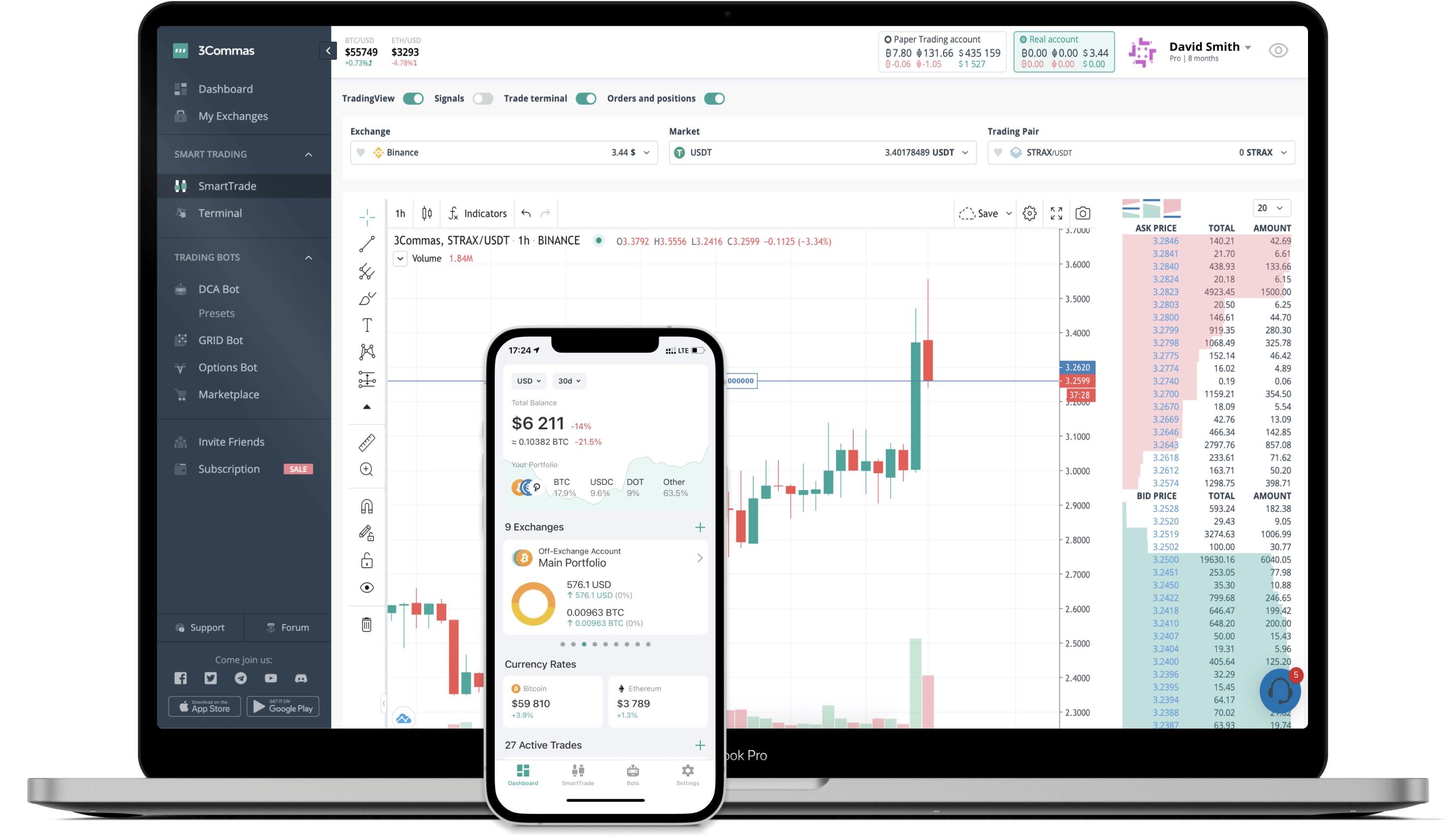LESS to INR Converter

Less Network
PriceINR 1.43-1.44%
VolumeINR 1,789
How it works

The 3Commas currency calculator allows you to convert a currency from Less Network (LESS) to Indian Rupee (INR) in just a few clicks at live exchange rates.

Simply enter the amount of Less Network you wish to convert to INR and the conversion amount automatically populates. You can also use our Prices Calculator Table to calculate how much your currency is worth in other denominations, i.e. .1 LESS, .5 LESS, 1 LESS, 5 LESS, or even 10 LESS.

LESS to INR Exchange Calculator

The chart displays Less Network price changes in INR.

The ultimate tools for crypto traders to maximize trading profits while minimizing risk and loss.Latest LESS to INR price calculator

Less Network market price is updated every three minutes and is automatically displayed in INR. Below are the most popular denominations to convert to INR.

Less Network
Indian Rupee
0.01
LESS
0.01
INR
0.1
LESS
0.14
INR
1
LESS
1.43
INR
2
LESS
2.86
INR
3
LESS
4.29
INR
5
LESS
7.15
INR
10
LESS
14.30
INR
25
LESS
35.75
INR
50
LESS
71.50
INR
100
LESS
143.00
INR
250
LESS
357.50
INR
500
LESS
715.00
INR
1000
LESS
1430.00
INR
2500
LESS
3575.00
INR
Indian Rupee
Less Network
0.01
INR
0.00699301
LESS
0.1
INR
0.06993007
LESS
1
INR
0.69930070
LESS
2
INR
1.39860140
LESS
3
INR
2.09790210
LESS
5
INR
3.49650350
LESS
10
INR
6.99300699
LESS
25
INR
17.48251748
LESS
50
INR
34.96503497
LESS
100
INR
69.93006993
LESS
250
INR
174.82517483
LESS
500
INR
349.65034965
LESS
1000
INR
699.30069930
LESS
2500
INR
1748.25174825
LESS

Top Currencies

The price of Less Network in other fiat and cryptocurrencies is based on the corresponding exchange rate of those currencies to the Indian Rupee.

FAQ

How much is 1 Less Network in Indian Rupee?

Less Network price in INR is constantly changing.

At this moment, 1 Less Network equals 1.43 INR

How do I calculate the conversion from LESS to INR?

The 3Commas Less Network Calculator allows you to easily calculate the conversion price of LESS to INR by simply entering the amount of Less Network in the corresponding field and will automatically convert the value in Indian Rupee (INR).

You can also use our Less Network price table above to check the latest Less Network price in major fiat and crypto currencies.

How can I convert Less Network to INR?

The most common way of converting LESS to INR is by using a Crypto Exchange or a P2P (person-to-person) exchange platform like LocalBitcoins, etc.